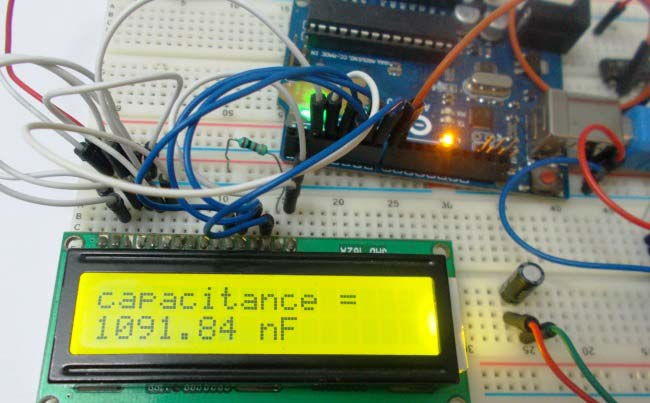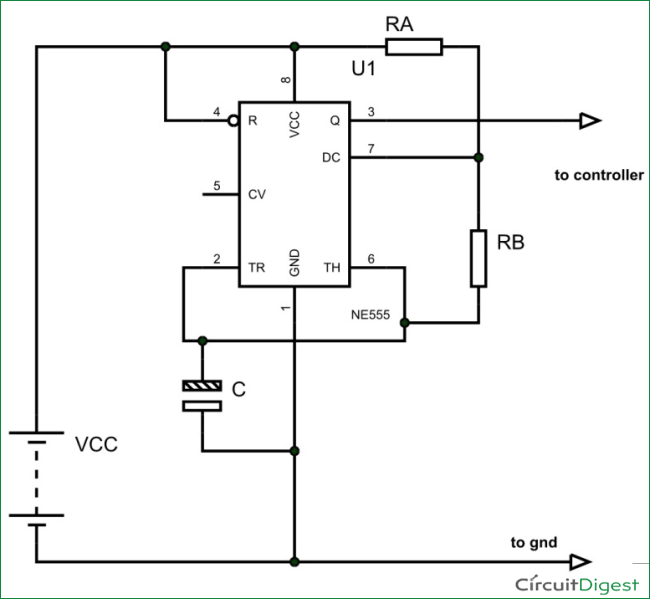# Capacitance Meter using Arduino

When we come across circuit boards which are previously designed or we take out one from old TV or computer, in attempt to repair it. And sometimes we need to know the capacitance of particular capacitor in the board to eliminate the fault. Then we face a problem in getting the exact value of capacitor from the board especially if it is a Surface Mount Device. We can buy equipment for measuring the capacitance, but all these devices are costly and not for everyone. With that in mind we are going to design a simple Arduino Capacitance Meter to measure the capacitance of unknown capacitors.

This meter can be easily made and also cost effective. We are going to make Capacitance Meter using Arduino Uno, Schmitt trigger gate and 555 IC timer.

### Required Components:

• 555 timer IC
• IC 74HC14 Schmitt trigger gate or NOT gate.
• 1K Ω resistor (2 pieces), 10KΩ resistor
• 100nF capacitor, 1000µF capacitor
• 16*2 LCD,### Circuit Explanation:

The circuit diagram of the Capacitance Meter using Arduino is shown in below figure. Circuit is simple, a LCD is interfaced with Arduino to display the measured Capacitance of capacitor. A Square wave Generator Circuit (555 in Astable mode) is connected to Arduino, where we have connected the Capacitor whose capacitance needs to be measured. A Schmitt trigger gate (IC 74LS14) is used to ensure that only rectangular wave is fed to Arduino. For filtering the noise we have added couple of capacitors across power.

This circuit can accurately measure capacitances in range 10nF to 10uF.### 555 Timer IC Based Square Wave Generator:

First of all we will talk about 555 Timer IC based square wave generator, or should I say 555 Astable Multivibrator.  We know that the capacitance of a capacitor cannot be measured directly in a digital circuit, in other words the UNO deals with digital signals and it cannot measure capacitance directly. So we use 555 square wave generator circuit for linking the capacitor to digital world.

Simply speaking, the timer provides square wave output whose frequency directly implicates to the capacitance connected to it. So first we get the square wave signal whose frequency is the representative of the capacitance of the unknown capacitor, and feed this signal to UNO for getting the appropriate value.The output signal frequency depends on RA, RB resistors and capacitor C. The equation is given as,

Frequency (F) = 1/ (Time period) = 1.44/ ((RA+RB*2)*C).

Here RA and RB are resistance values and C is capacitance value. By putting the resistance and capacitance values in above equation we get the frequency of output square wave.

We are going to connect 1KΩ as RA and 10KΩ as RB. So the formula becomes,

Frequency (F) = 1/ (Time period) = 1.44/ (21000*C).

By rearranging the terms we have,

Capacitance C = 1.44/ (21000*F)

In our Program Code (see below), for getting the capacitance value accurately we have calculated the result in nF by multiplying the obtained results (in farads) with “1000000000”.  Also we have used ‘20800’ instead of 21000, because the accurate resistances of RA and RB are 0.98K and 9.88K.

READ  Aquameterduino: Water meter plus Arduino

So if we know the frequency of the square wave we can get the capacitance value.

### Schmitt Trigger Gate:

The signals generated by the timer circuit are not completely safe to be directly given to the Arduino Uno. With the sensitivity of UNO in mind, we use Schmitt trigger gate. Schmitt trigger gate is a digital logic gate.

This gate provides OUTPUT based on INPUT voltage level. A Schmitt Trigger has a THERSHOLD voltage level, when the INPUT signal applied to the gate has a voltage level higher than the THRESHOLD of the logic gate, OUTPUT goes HIGH.

Read More:  Capacitance Meter using Arduino

#### This Post / Project can also be found using search terms:

• arduino capacitance meter in proteus
• arduino capacitor meter
• capacitor meter using arduino